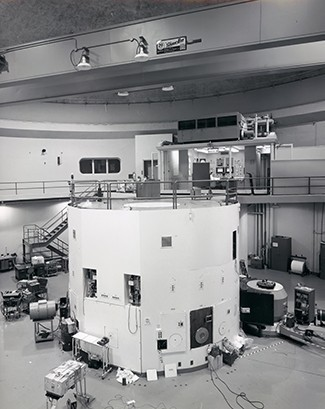## Introduction to Exponential and Logarithmic Models

### Learning Outcomes

By the end of this lesson, you will be able to:

• Graph exponential growth and decay functions.
• Solve problems involving radioactive decay, carbon dating, and half life.
• Use Newton’s Law of Cooling.
• Use a logistic growth model.
• Choose an appropriate model for data.
• Use a graphing utility to create an exponential regression from a set of data.

## What you’ll learn to do: Graph and solve real world problems involving data that follow logarithmic or exponential growth and decay trendsA nuclear research reactor inside the Neely Nuclear Research Center on the Georgia Institute of Technology campus. (credit: Georgia Tech Research Institute)

We have already explored some basic applications of exponential and logarithmic functions. In this section, we explore some important applications in more depth including radioactive isotopes and Newton’s Law of Cooling.

## Contribute!

Did you have an idea for improving this content? We’d love your input.